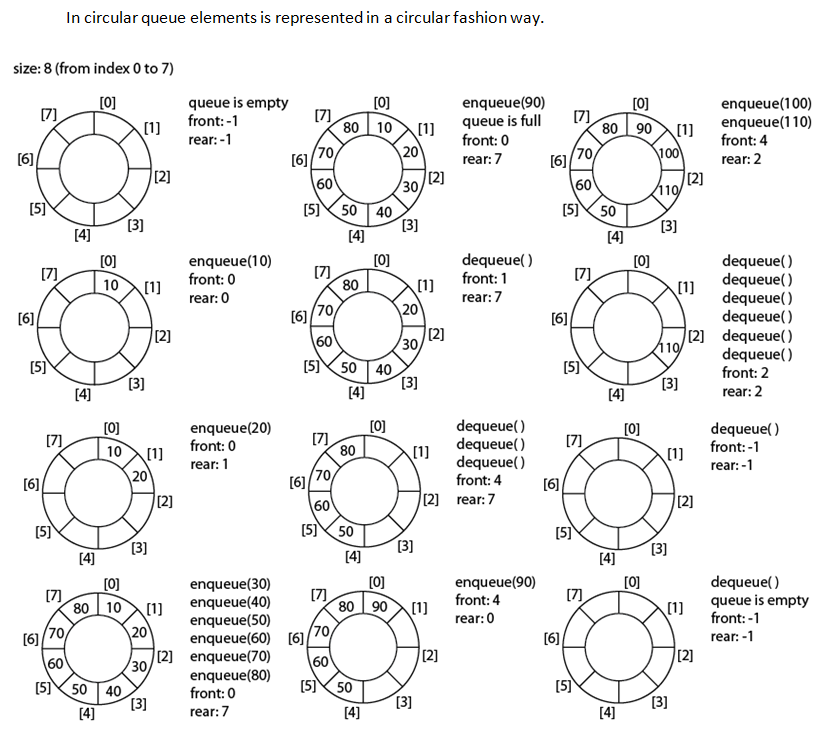# Queue

QueueQueue is a linear data structure in which data can be added to one end and retrieved from the other. Just like the queue of the real world, the data that goes first into the queue is the first one to be retrieved. That is why queues are sometimes called as First-In-First-Out data structure.

In case of stack, we saw that data is inserted both from one end but in case of Queues; data is added to one end (known as REAR) and retrieved from the other end (known as FRONT).

The data first added is the first one to be retrieved while in case of queues the data last added is the first one to be retrieved.

Few points regarding Queue:

1.Queue: It is a linear data structure; linked lists and arrays can represent it.

2.Rear: A variable stores the index number in the array at which the new data will be added (in the queue).

3.Front: It is a variable storing the index number in the array where the data will be retrieved.

// Array Implementation of Queue

#include <iostream.h>
#define MAX 5
#include <stdlib.h>
int queue[MAX];
int front , rear;
void insertQ();
void deleteQ();
void insertQ()

{
int value;
cout<<endl<<“Enter the element to be inserted in queue\n”;
cin>>value;
if(rear < MAX-1)
{
rear= rear +1;
queue[rear] = value;
}
else
{
cout<<“The queue is full \n”;
exit(1);
}
}
void deleteQ()

{   int value;
if(front == rear)
{
cout<<“The queue is empty \n”;
exit(1);
}
front = front + 1;
value = queue[front];
cout<<endl<<“The value deleted is “<<value;
}
int main()
{
int n;
front=rear=-1;
do
{
do
{
insertQ();
cout<<“Enter 1 to continue and 0 for exit\n”;
cin>>n;
}while(n == 1);

cout<<endl<<“Enter 1 to delete an element and 0 for exit” ;
cin>>n;
while( n == 1)
{
deleteQ();
cout<<endl<<“Enter 1 to delete an element and 0 for exit”;
cin>>n;
}
cout<<endl<<“Enter 1 to continue and 0 for exit\n”;
cin>>n;
} while(n == 1);
return 0;
}

// Linked Implementation of Queue data Structure

#include<iostream.h>
#include<stdlib.h>

struct node
{
int data;
};

node *front=NULL;
node *rear=NULL;
void insertQ();
void deleteQ();
void display();

int main()
{
int n;
do
{
cin>>n;
switch(n)
{
case 1:
insertQ();
break;
case 2:
deleteQ();
break;
case 3:
display();
break;
case 4:
break;
default:
cout<<“Invalid choice\n”;
break;
}
}while(n!=4);
return 0;
}
void insertQ()
{
int item;
node *temp;
cout<<“Enter the item\n”;
cin>>item;
temp= new node();
temp->data=item;
if(rear==NULL)
{
front=temp;
rear=temp;
}
else
{
rear=temp;
}
}
void deleteQ()
{
int item;
if(front==NULL)
cout<<“Queue is empty\n”;
else
{
item=front->data;
cout<<“The element deleted = \n”<<item;
}
if(front==rear)
{
front=NULL;
rear=NULL;
}
else
}
void display()
{
node *ptr;

if(front==NULL)
cout<<“Queue is empty\n”;
else
{
ptr=front;
cout<<“The elements of the queue are :”;
while(ptr!=NULL)
{
cout<<ptr->data<<endl;
}
}
}

Circular Queue

In circular queue, the insertion of a new element is performed at the very first location of the queue if the last location of the queue is full, in which the first element comes  just after the last element.

It overcomes the problem of unutilized space in leaner queues, when it is  implemented as arrays.

Insertion

Rear = (rear+1)%Maxsize

Algorithm Steps:

Step 1:

• create and set the variables front,rear,MAXSIZE,cq[]

step 2:

• Read the circular queue operation type.

step 3:

• If  operation type is Insertion below steps are executed.
• Assign rear=rear%MAXSIZE.
• if front equal to (rear+1)%MAXSIZE then display queue is   overflow.
• if front equal to -1 then assign front=rear=0.
• Otherwise assign rear=(rear+1)%MAXSIZE and read queue data
Assign cq[rear] as data.(i.e. cq[rear]=data).

step 4:

• If operation type is Deletion below steps are executed.
• Check front=-1 then display queue is underflow.
• Set temp as cq[front] (i.e. temp=ca[front]).
• Check front equal to rear if it is true then assign
• front=rear=-1(Move the front to beginning)
• Assign front=(front+1)%MAXSIZE.//Program for Circular Queue implementation through Array
#include <iostream.h>
#include<ctype.h>
#include<stdlib.h>
#include<conio.h>

#define MAXSIZE 5
int cq[MAXSIZE];
int front,rear;
int main()
{
void del();
int will=1,i,num;
front = -1;
rear = -1;
//clrscr();
cout<<“\nProgram for Circular Queue through array”;
while(1)
{
cin>>will;
switch(will)
{
case 1:
cout<<“\n\nENTER THE QUEUE ELEMENT : “;
cin>>num;
break;
case 2:
del();
break;
case 3:
exit(0);
default:
cout<<“\n\nInvalid Choice . “;
}
} //end of  outer while
return 0;
}               //end of main
{
//rear++;
//rear= (rear%MAXSIZE);
if(front ==(rear+1)%MAXSIZE)
{
cout<<“\n\nCIRCULAR QUEUE IS OVERFLOW”;
}
else
{
if(front==-1)
front=rear=0;
else
rear=(rear+1)%MAXSIZE;
cq[rear]=item;
cout<<“\n\nRear = “<<rear<<”  Front = “<<front;
}
}
void del()
{
int a;
if(front == -1)
{
cout<<“\nCIRCULAR QUEUE IS UNDERFLOW”;
}
else
{
a=cq[front];
if(front==rear)
front=rear=-1;
else
front = (front+1)%MAXSIZE;
cout<<“\n\nDELETED ELEMENT FROM QUEUE IS :  “<<a;
cout<<“\n\nRear =   “<<rear<<”  Front = “<<rear;

}
}